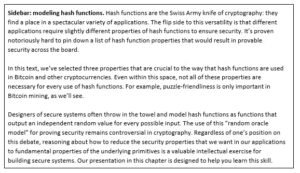# Cryptographic Hash Functions

The first cryptographic primitive that we’ll need to understand is a cryptographic hash function. A

hash function is a mathematical function with the following three properties:

• Its input can be any string of any
• It produces a fixed size For the purpose of making the discussion in this chapter concrete, we will assume a 256‐bit output size. However, our discussion holds true for any output size as long as it is sufficiently large.
• It is efficiently Intuitively this means that for a given input string, you can figureout what the output of the hash function is in a reasonable amount of time. More technically, computing the hash of an n‐bit string should have a running time that is O(n).

Those properties define a general hash function, one that could be used to build a data structure such as a hash table. We’re going to focus exclusively on cryptographic hash functions. For a hash function to be cryptographically secure, we’re going to require that it has the following three additional properties: (1) collision‐resistance, (2) hiding, (3) puzzle‐friendliness.

We’ll look more closely at each of these properties to gain an understanding of why it’s useful to have a function that behaves that way. The reader who has studied cryptography should be aware that the treatment of hash functions in this book is a bit different from a standard cryptography textbook. The puzzle‐friendliness property, in particular, is not a general requirement for cryptographic hash functions, but one that will be useful for cryptocurrencies specifically.

Property 1: Collision‐resistance. The first property that we need from a cryptographic hash function is that it’s collision‐resistant. A collision occurs when two distinct inputs produce the same output. A hash function H(.) is collision‐resistant if nobody can find a collision. Formally: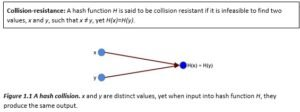Notice that we said nobody can find a collision, but we did not say that no collisions exist. Actually, we know for a fact that collisions do exist, and we can prove this by a simple counting argument. The input space to the hash function contains all strings of all lengths, yet the output space contains only strings of a specific fixed length. Because the input space is larger than the output space (indeed, the input space is infinite, while the output space is finite), there must be input strings that map to the same output string. In fact, by the Pigeonhole Principle there will necessarily be a very large number of possible inputs that map to any particular output.Now, to make things even worse, we said that it has to be impossible to find a collision. Yet, there are methods that are guaranteed to find a collision. Consider the following simple method for finding a collision for a hash function with a 256‐bit output size: pick 2256 + 1 distinct values, compute the hashes of each of them, and check if there are any two outputs are equal. Since we picked more inputs than possible outputs, some pair of them must collide when you apply the hash function.

The method above is guaranteed to find a collision. But if we pick random inputs and compute the hash values, we’ll find a collision with high probability long before examining 2256 + 1 inputs. In fact, if we randomly choose just 2130 + 1 inputs, it turns out there’s a 99.8% chance that at least two of them are going to collide. The fact that we can find a collision by only examining roughly the square root of the number of possible outputs results from a phenomenon in probability known as the birthday paradox. In the homework questions at the end of this chapter, we will examine this in more detail.

This collision‐detection algorithm works for every hash function. But, of course, the problem with it is that this takes a very, very long time to do. For a hash function with a 256‐bit output, you would have to compute the hash function 2256 + 1 times in the worst case, and about 2128 times on average. That’s of course an astronomically large number — if a computer calculates 10,000 hashes per second, it would take more than one octillion (1027) years to calculate 2128 hashes! For another way of thinking about this, we can say that, if every computer ever made by humanity was computing since the beginning of the entire universe, up to now, the odds that they would have found a collision is still infinitesimally small. So small that it’s way less than the odds that the Earth will be destroyed by a giant meteor in the next two seconds.

We have thus seen a general but impractical algorithm to find a collision for any hash function. A  more difficult question is: is there some other method that could be used on a particular hash function in order to find a collision? In other words, although the generic collision detection algorithm is        not feasible to use, there still may be some other algorithm that can efficiently find a collision for a specific hash function.

Consider, for example, the following hash function:

H(x) = x mod 2256

This function meets our requirements of a hash function as it accepts inputs of any length, returns a fixed sized output (256 bits), and is efficiently computable. But this function also has an efficient method for finding a collision. Notice that this function just returns the last 256 bits of the input. One collision then would be the values 3 and 3 + 2256. This simple example illustrates that even though our generic collision detection method is not usable in practice, there are at least some hash functions for which an efficient collision detection method does exist.

Yet for other hash functions, we don’t know if such methods exist. We suspect that they are collision resistant. However, there are no hash functions proven to be collision‐resistant. The cryptographic hash functions that we rely on in practice are just functions for which people have tried really, really hard to find collisions and haven’t yet succeeded. In some cases, such as the old MD5 hash function, collisions were eventually found after years of work, leading the function to be deprecated and phased out of practical use. And so we choose to believe that those are collision resistant.

Application: Message digests Now that we know what collision‐resistance is, the logical question is: What is collision‐resistance useful for? Here’s one application: If we know that two inputs x and y to a collision‐resistant hash function H are different, then it’s safe to assume that their hashes H(x) and H(y) are different — if someone knew an x and y that were different but had the same hash, that would violate our assumption that H is collision resistant.

Collision‐free hashes provide an elegant and efficient solution to this problem. Alice just needs to remember the hash of the original file. When she later downloads the file from SecureBox, she computes the hash of the downloaded file and compares it to the one she stored. If the hashes are

the same, then she can conclude that the file is indeed the one she uploaded, but if they are different, then Alice can conclude that the file has been tampered with. Remembering the hash thus allows her to detect accidental corruption of the file during transmission or on SecureBox’s servers, but also intentional modification of the file by the server. Such guarantees in the face of potentially malicious behavior by other entities are at the core of what cryptography gives us.

The hash serves as a fixed length digest, or unambiguous summary, of a message. This gives us a very efficient way to remember things we’ve seen before and recognize them again. Whereas the entire file might have been gigabytes long, the hash is of fixed length, 256‐bits for the hash function in our example. This greatly reduces our storage requirement. Later in this chapter and throughout the book, we’ll see applications for which it’s useful to use a hash as a message digest.

Property 2: Hiding The second property that we want from our hash functions is that it’s hiding. The hiding property asserts that if we’re given the output of the hash function y = H(x), there’s no feasible way to figure out what the input, x, was. The problem is that this property can’t be true in the stated form. Consider the following simple example: we’re going to do an experiment where we flip a coin. If the result of the coin flip was heads, we’re going to announce the hash of the string “heads”. If the result was tails, we’re going to announce the hash of the string “tails”.

We then ask someone, an adversary, who didn’t see the coin flip, but only saw this hash output, to figure out what the string was that was hashed (we’ll soon see why we might want to play games like this). In response, they would simply compute both the hash of the string “heads” and the hash of the string “tails”, and they could see which one they were given. And so, in just a couple steps, they can figure out what the input was.

The adversary was able to guess what the string was because there were only two possible values of x, and it was easy for the adversary to just try both of them. In order to be able to achieve the hiding property, it needs to be the case that there’s no value of x which is particularly likely. That is, x has to

be chosen from a set that’s, in some sense, very spread out. If x is chosen from such a set, this method of trying a few values of x that are especially likely will not work.

The big question is: can we achieve the hiding property when the values that we want do not come from a spread‐out set as in our “heads” and “tails” experiment? Fortunately, the answer is yes! So perhaps we can hide even an input that’s not spread out by concatenating it with another input that is spread out. We can now be slightly more precise about what we mean by hiding (the double vertical bar ‖ denotes concatenation).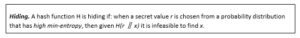In information‐theory, min‐entropy is a measure of how predictable an outcome is, and high min‐entropy captures the intuitive idea that the distribution (i.e., random variable) is very spread out. What that means specifically is that when we sample from the distribution, there’s no particular value that’s likely to occur. So, for a concrete example, if r is chosen uniformly from among all of the strings that are 256 bits long, then any particular string was chosen with probability 1/2256, which is an infinitesimally small value.

Application: Commitments. Now let’s look at an application of the hiding property. In particular, what we want to do is something called a commitment. A commitment is the digital analog of taking a value, sealing it in an envelope, and putting that envelope out on the table where everyone can see it. When you do that, you’ve committed yourself to what’s inside the envelope. But you haven’t opened it, so even though you’ve committed to a value, the value remains a secret from everyone else. Later, you can open the envelope and reveal the value that you committed to earlier.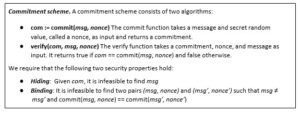To use a commitment scheme, we first need to generate a random nonce. We then apply the commit function to this nonce together with msg, the value being committed to, and we publish the commitment com. This stage is analogous to putting the sealed envelope on the table. At a later point, if we want to reveal the value that they committed to earlier, we publish the random nonce that we used to create this commitment, and the message, msg. Now, anybody can verify that msg was indeed the message committed to earlier. This stage is analogous to opening up the envelope.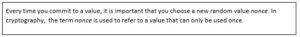The two security properties dictate that the algorithms actually behave like sealing and opening an envelope. First, given com, the commitment, someone looking at the envelope can’t figure out what the message is. The second property is that it’s binding. This ensures that when you commit to what’s in the envelope, you can’t change your mind later. That is, it’s infeasible to find two different messages, such that you can commit to one message, and then later claim that you committed to another.

So how do we know that these two properties hold? Before we can answer this, we need to discuss how we’re going to actually implement a commitment scheme. We can do so using a cryptographic hash function. Consider the following commitment scheme:

commit(msg, nonce) := H(nonce msg) where nonce is a random 256‐bit value

To commit to a message, we generate a random 256‐bit nonce. Then we concatenate the nonce and the message and return the hash of this concatenated value as the commitment. To verify, someone will compute this same hash of the nonce they were given concatenated with the message. And they will check whether that’s equal to the commitment that they saw.

Take another look at the two properties that we require of our commitment schemes. If we substitute the instantiation of commit and verify as well as H(nonce msg) for com, then these properties

become:

• Hiding: Given H(nonce msg), it is infeasible to find msg
• Binding: It is infeasible to find two pairs (msg, nonce) and (msg’, nonce’) such that msg msg’

and H(nonce msg) == H(nonce’ msg’)

The hiding property of commitments is exactly the hiding property that we required for our hash functions. If key was chosen as a random 256‐bit value then the hiding property says that if we hash the concatenation of key and the message, then it’s infeasible to recover the message from the hash output. And it turns out that the binding property is implied by1 the collision‐resistant property of the underlying hash function. If the hash function is collision‐resistant, then it will be infeasible to find distinct values msg and msg’ such that H(nonce msg) = H(nonce’ msg’) since such values would indeed be a collision.

Therefore, if H is a hash function that is collision‐resistant and hiding, this commitment scheme will work, in the sense that it will have the necessary security properties.

Property 3: Puzzle friendliness. The third security property we’re going to need from hash functions is that they are puzzle‐friendly. This property is a bit complicated. We will first explain what the technical requirements of this property are and then give an application that illustrates why this property is useful.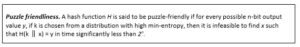Intuitively, what this means is that if someone wants to target the hash function to come out to some particular output value y, that if there’s part of the input that is chosen in a suitably randomized way, it’s very difficult to find another value that hits exactly that target.

Application: Search puzzle. Now, let’s consider an application that illustrates the usefulness of this property. In this application, we’re going to build a search puzzle, a mathematical problem which requires searching a very large space in order to find the solution. In particular, a search puzzle has no shortcuts. That is, there’s no way to find a valid solution other than searching that large space.

The reverse implications do not hold. That is, it’s possible that you can find collisions, but none of them are of the form H(nonce msg) == H(nonce’ msg’). For example, if you can only find a collision in which two distinct nonces generate the same commitment for the same message, then the commitment scheme is still binding, but the underlying hash function is not collision‐resistant.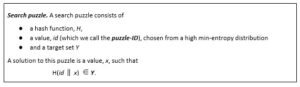The intuition is this: if H has an n‐bit output, then it can take any of 2n values. Solving the puzzle requires finding an input so that the output falls within the set Y, which is typically much smaller than the set of all outputs. The size of Y determines how hard the puzzle is. If Y is the set of all n‐bit strings the puzzle is trivial, whereas if Y has only 1 element the puzzle is maximally hard. The fact that the puzzle id has high min‐entropy ensures that there are no shortcuts. On the contrary, if a particular value of the ID were likely, then someone could cheat, say by pre‐computing a solution to the puzzle with that ID.

If a search puzzle is puzzle‐friendly, this implies that there’s no solving strategy for this puzzle which is much better than just trying random values of x. And so, if we want to pose a puzzle that’s difficult to solve, we can do it this way as long as we can generate puzzle‐IDs in a suitably random way. We’re going to use this idea later when we talk about Bitcoin mining, which is a sort of computational puzzle.

SHA‐256. We’ve discussed three properties of hash functions, and one application of each of those. Now let’s discuss a particular hash function that we’re going to use a lot in this book. There are lots of hash functions in existence, but this is the one Bitcoin uses primarily, and it’s a pretty good one to use. It’s called SHA‐256.

Recall that we require that our hash functions work on inputs of arbitrary length. Luckily, as long as  we can build a hash function that works on fixed‐length inputs, there’s a generic method to convert it into a hash function that works on arbitrary‐length inputs. It’s called the Merkle‐Damgard transform. SHA‐256 is one of a number of commonly used hash functions that make use of this method. In common terminology, the underlying fixed‐length collision‐resistant hash function is called the compression function. It has been proven that if the underlying compression function is collision resistant, then the overall hash function is collision resistant as well.

The Merkle‐Damgard transform is quite simple. Say the compression function takes inputs of length m and produces an output of a smaller length n. The input to the hash function, which can be of any size, is divided into blocks of length m‐n. The construction works as follows: pass each block together with the output of the previous block into the compression function. Notice that input length will then be (m‐n) + n = m, which is the input length to the compression function. For the first block, to which

there is no previous block output, we instead use an Initialization Vector (IV). This number is reused for every call to the hash function, and in practice you can just look it up in a standards document. The last block’s output is the result that you return.

SHA‐256 uses a compression function that takes 768‐bit input and produces 256‐bit outputs. The block size is 512 bits. See Figure 1.3 for a graphical depiction of how SHA‐256 works.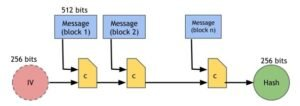Figure 1.3: SHA‐256 Hash Function (simplified). SHA‐256 uses the Merkle‐Damgard transform to turn a fixed‐length collision‐resistant compression function into a hash function that accepts arbitrary‐length inputs. The input is “padded” so that its length is a multiple of 512 bits.

We’ve talked about hash functions, cryptographic hash functions with special properties, applications of those properties, and a specific hash function that we use in Bitcoin. In the next section, we’ll discuss ways of using hash functions to build more complicated data structures that are used in distributed systems like Bitcoin.